## 场强重整化

### 两点关联函数的结构

$\langle \Omega|T\phi(x)\phi(y)|\Omega\rangle$

$\langle 0|T\phi(x)\phi(y)|0\rangle = D_F(x-y) = \int \frac{d^4p}{(2\pi)^4}\frac{i}{p^2-m^2+i\epsilon}e^{-ip(x-y)}$

$p^2 = m^2$ 为该传播子的极点，对应一个质量为 $m$ 的自由粒子态。

$(1)_{1-particle} = \int \frac{d^3p}{(2\pi)^3}\frac{1}{2E_{\bm{p}}}|\bm{p}\rangle\langle \bm{p}|$

$1 = |\Omega\rangle\langle\Omega| + \sum_{\lambda}\int \frac{d^3p}{(2\pi)^3} \frac{1}{2E_{\bm{p}}(\lambda)} |\lambda_{\bm{p}}\rangle\langle\lambda_{\bm{p}}|$

$\langle\Omega|\phi(x)\phi(y)|\Omega\rangle = \sum_{\lambda}\int \frac{d^3p}{(2\pi)^3} \frac{1}{2E_{\bm{p}}(\lambda)}\langle\Omega| \phi(x)|\lambda_{\bm{p}}\rangle\langle\lambda_{\bm{p}}| \phi(y)|\Omega\rangle\tag{1}$

\begin{aligned} \langle\Omega|\phi(x)|\lambda_{\bm{p}}\rangle &= \langle\Omega| e^{iP\cdot x}\phi(0)e^{-iP\cdot x}|\lambda_{\bm{p}}\rangle\\ &= \langle\Omega|\phi(0)|\lambda_{\bm{p}}\rangle e^{-ip\cdot x}|_{p^0 = E_{\bm{p}}}\\ &= \langle\Omega|\phi(0)|\lambda_{0}\rangle e^{-ip\cdot x}|_{p^0 = E_{\bm{p}}}\\ \end{aligned}

\begin{aligned} \langle \Omega|\phi(x)\phi(y)|\Omega\rangle &= \sum_{\lambda}\int \frac{d^4p}{(2\pi)^4} \frac{i}{p^2-m_{\lambda}^2 + i\epsilon}e^{-ip\cdot(x-y)}|\langle\Omega|\phi(0)|\lambda_0\rangle|^2\\ &= \int_{0}^{\infty} \frac{dM^2}{2\pi}\rho(M^2)D_F(x-y;M^2)\\ \end{aligned}\tag{2}

$\rho(M^2) = \sum_{\lambda} (2\pi)\delta(M^2 - m_{\lambda}^2) |\langle\Omega|\phi(0)|\lambda_0\rangle|^2\tag{3}$

$\rho(M^2) = 2\pi \delta(M^2-m^2)$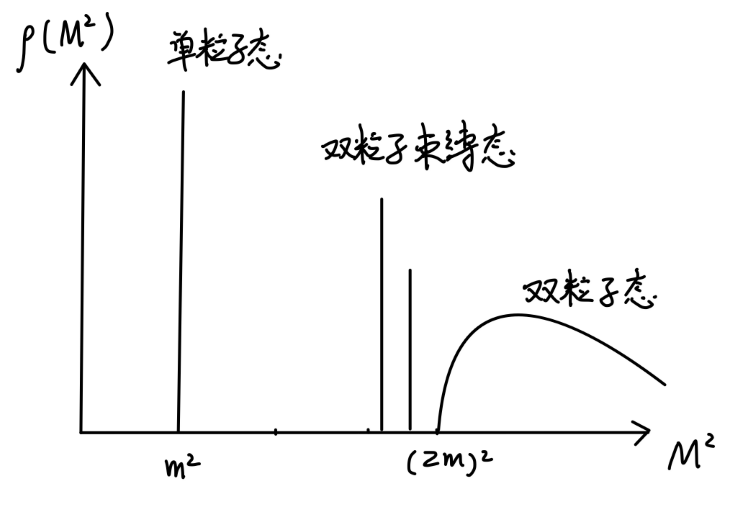$\rho(M^2) = 2\pi \delta(M^2-m^2)\cdot Z + (nothing\ else\ until\ M^2 \gtrsim (2m)^2)$

\begin{aligned} \int d^4x e^{ip\cdot x}\langle\Omega|T\phi(x)\phi(0)|\Omega\rangle &= \int_{0}^{\infty} \frac{dM^2}{2\pi} \rho(M^2) \frac{i}{p^2-M^2+i\epsilon}\\ &=\frac{iZ}{p^2 - m^2 + i\epsilon} + \int_{\sim 4m^2}^{\infty} \frac{dM^2}{2\pi} \rho(M^2) \frac{i}{p^2-M^2+i\epsilon}\\ \end{aligned}\tag{4}

$\langle 0|T\phi(x)\phi(0)|0\rangle = \frac{i}{p^2-m^2+i\epsilon}$

\begin{aligned} \int d^4x e^{ip\cdot x}\langle\Omega|T\psi(x)\bar{\psi}(0)|\Omega\rangle &= \frac{iZ_2\sum_s u^s(p)\bar{u}^s(p)}{p^2-m^2+i\epsilon} + \cdots\\ &= \frac{iZ_2(\cancel{p}+m)}{p^2-m^2+i\epsilon} + \cdots\\ \end{aligned}

$\langle \Omega|\psi(0)|p,s\rangle = \sqrt{Z_2}u^s(p)$

### 电子自能$\frac{i\cancel{p}+m_0}{p^2-m_0^2 + i\epsilon}$

$\frac{i(\cancel{p}+m_0)}{p^2-m_0^2}[-i\Sigma_2(p)]\frac{i(\cancel{p}+m_0)}{p^2-m_0^2}$

$-i\Sigma_2(p) = (-ie)^2 \int \frac{d^4k}{(2\pi)^4}\gamma^{\mu}\frac{i(\cancel{k}+m_0)}{k^2-m_0^2+i\epsilon} \gamma_{\mu} \frac{-i}{(p-k)^2 - \mu^2 +i\epsilon}$

$\frac{1}{k^2-m_0^2 + i\epsilon}\frac{1}{k^2-\mu^2 + i\epsilon} = \int_{0}^{1}dx \frac{1}{[k^2 - 2xk\cdot p +xp^2 -x\mu^2 -(1-x)m_0^2 + i\epsilon]^2}$

$-i\Sigma_2(p) = -e^2 \int_{0}^{1}dx\int \frac{d^4l}{(2\pi)^4} \frac{-2x\cancel{p} + 4m_0}{(l^2-\Delta + i\epsilon)}$

$l \equiv k-xp\quad \Delta = -x(1-x)p^2 + x\mu^2 + (1-x)m_0^2$

$\frac{1}{(k-p)^2 - \mu^2 +i\epsilon} \rightarrow \frac{1}{(p-k)^2 - \mu^2 + i\epsilon} - \frac{1}{(p-k)^2 - \Lambda^2 + i\epsilon}$

$\Sigma_2 = \frac{\alpha}{2\pi} \int_{0}^{1}dx(2m_0-x\cancel{p})\log (\frac{x\Lambda^2}{(1-x)m_0^2 + x\mu^2 -x(1-x)p^2})\tag{5}$

$(1-x)m_0^2 + x\mu^2 -x(1-x)p^2 = 0$

$x = \frac{1}{2} + \frac{m_0^2}{2p^2} - \frac{\mu^2}{2p^2} \pm \frac{1}{2p^2}\sqrt{[p^2-(m_0+\mu)^2][p^2-(m_0-\mu)^2]}$

$p^2 \geqslant (m_0+\mu)^2$ 时，我们得到两个解 $x\in[0,1]$。此时是存在 branch cut 的。这对应产生一个电子加一个光子的两粒子态。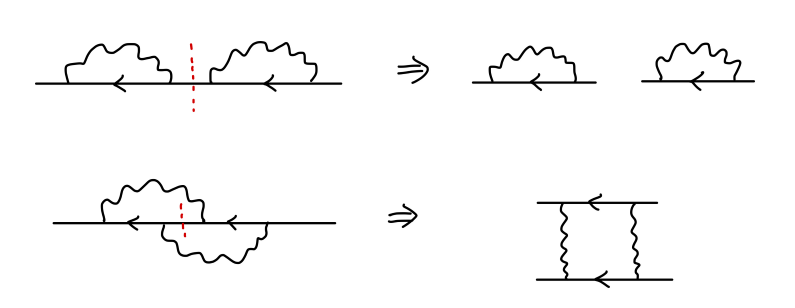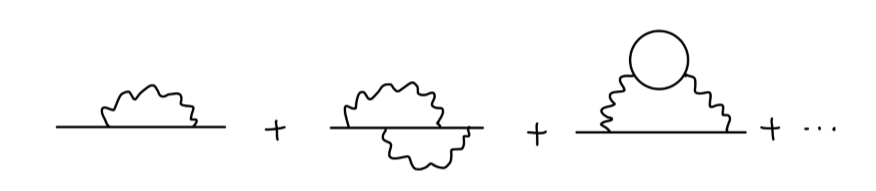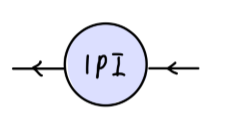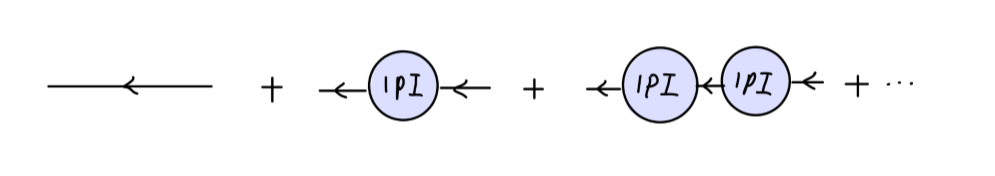\begin{aligned} &\int d^4x \langle\Omega|T\psi(x)\bar{\psi}(0)|\Omega\rangle e^{ip\cdot x}\\ &= \frac{i(\cancel{p}+m_0)}{p^2-m_0^2} + \frac{i(\cancel{p}+m_0)}{p^2-m_0^2}(-i\Sigma)\frac{i(\cancel{p}+m_0)}{p^2-m_0^2} + \cdots\\ &= \frac{i}{\cancel{p}-m_0} + \frac{i}{\cancel{p}-m_0} (\frac{\Sigma(\cancel{p})}{\cancel{p}-m_0}) + \frac{i}{\cancel{p}-m_0} (\frac{\Sigma(\cancel{p})}{\cancel{p}-m_0})^2 + \cdots \\ &= \frac{i}{\cancel{p} - m_0 - \Sigma(\cancel{p})} \end{aligned}\tag{6}

$[\cancel{p} -m_0 - \Sigma(\cancel{p})]|_{\cancel{p} = m} = 0\tag{7}$

$(\cancel{p}-m)\cdot(1-\frac{d\Sigma}{d\cancel{p}}|_{\cancel{p} = m}) + \mathcal{O}((\cancel{p}-m)^2)$

$Z_2^{-1} = 1-\frac{d\Sigma}{d\cancel{p}}|_{\cancel{p} = m}\tag{8}$

$\delta m = m - m_0 = \Sigma_2(\cancel{p}=m) \approx \Sigma_2(\cancel{p}=m_0)$

\begin{aligned} \delta m &= \frac{\alpha}{2\pi}m_0 \int_{0}^{1}dx(2-x)\log (\frac{x\Lambda^2}{(1-x)^2m_0^2 + x\mu^2})\\ &\underset{\Lambda\rightarrow\infty}{\rightarrow} \frac{3\alpha}{4\pi}m_0 \log (\frac{\Lambda^2}{m_0^2})\\ \end{aligned}\tag{9}

\begin{aligned} \delta Z_2 &= Z_2 - 1\\ &= \frac{d\Sigma_2}{d\cancel{p}}|_{\cancel{p}=m}\\ &= \frac{\alpha}{2\pi}\int_{0}^{1}dx[-x\log \frac{x\Lambda^2}{(1-x)^2m^2+x\mu^2} + 2(2-x)\frac{x(1-x)m^2}{(1-x)^2m^2+x\mu^2}] \end{aligned}

\begin{aligned} \delta F_1(0) &= \frac{\alpha}{2\pi}\int_{0}^{1}dxdydz\delta(x+y+z-1)\\ &\quad \times [\log(\frac{z\Lambda^2}{(1-z)^2m^2+z\mu^2}) + \frac{(1-4z+z^2)m^2}{(1-z)^2m^2 + z\mu^2}]\\ &= \frac{\alpha}{2\pi}\int_{0}^{1}dz(1-z)(\log(\frac{z\Lambda^2}{(1-z)^2m^2+z\mu^2}) + \frac{(1-4z+z^2)m^2}{(1-z)^2m^2 + z\mu^2}) \end{aligned}

\begin{aligned} \int_{0}^{1}dz(1-2z)\log(\frac{\Lambda^2}{(1-z)^2m^2+z\mu^2}) &= -\int_{0}^{1}dz z(1-z)\frac{2(1-z)m^2-\mu^2}{(1-z)^2m^2 + z\mu^2}\\ &= \int_{0}^{1}dz[(1-z) - \frac{(1-z)(1-z^2)m^2}{(1-z)^2m^2+z\mu^2}]\\ \end{aligned}

\begin{aligned} \delta F_1(0) + \delta Z_2=&\frac{\alpha}{2\pi}\int_{0}^{1}dz[(1-2z)\log \frac{\Lambda^2}{(1-z)^2m^2+z\mu^2}+ (1-2z)\log z\\ &\quad + 2(2-z)\frac{z(1-z)m^2}{(1-z)^2m^2+z\mu^2} + \frac{(1-4z+z^2)m^2}{(1-z)^2m^2 + z\mu^2}]\\ &= \frac{\alpha}{2\pi}\int_{0}^{1}dz [(1-z) - \frac{(1-z)(1-z^2)m^2}{(1-z)^2m^2+z\mu^2}] + (1-2z)\log z\\ &\quad + 2(2-z)\frac{z(1-z)m^2}{(1-z)^2m^2+z\mu^2} + \frac{(1-4z+z^2)m^2}{(1-z)^2m^2 + z\mu^2}]\\ &=\frac{\alpha}{2\pi}\int_{0}^{1}dz(1-z + (1-2z)\log z)\\ &=\frac{\alpha}{2\pi} (z-z^2)\log z|_{0}^{1} = 0\\ \end{aligned}

$\delta F_1(0) + \delta Z_2=0\tag{10}$

\begin{aligned} \gamma^{\mu} \rightarrow \gamma^{\mu}F_1(q^2)Z_2 &\approx \gamma^{\mu}(1 + \delta F_1(q^2) + \delta Z_2)\\ &= \gamma^{\mu}(1+\delta F_1(q^2) - \delta F_1(0)) \\ \end{aligned}